1 Common Factor and Highest Common Factor My

• Slides: 35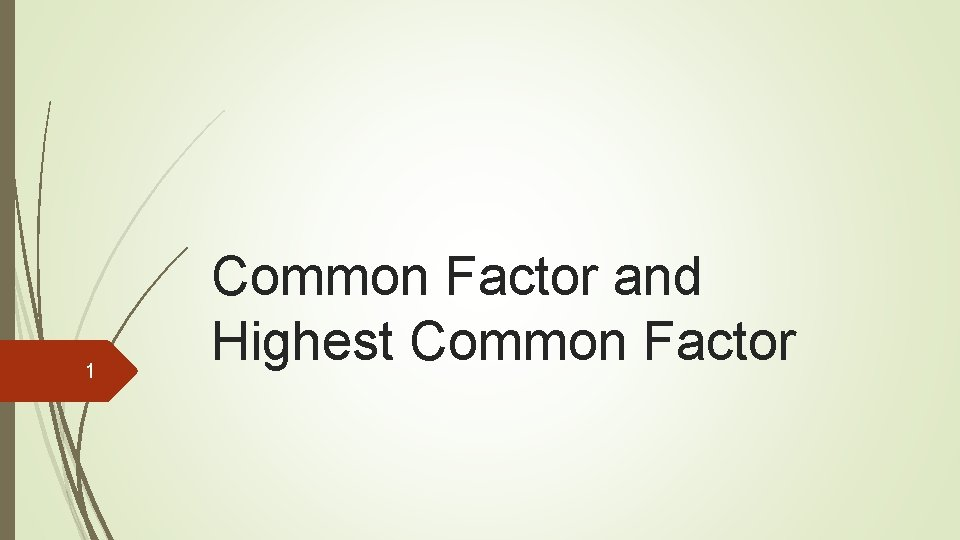1 Common Factor and Highest Common Factor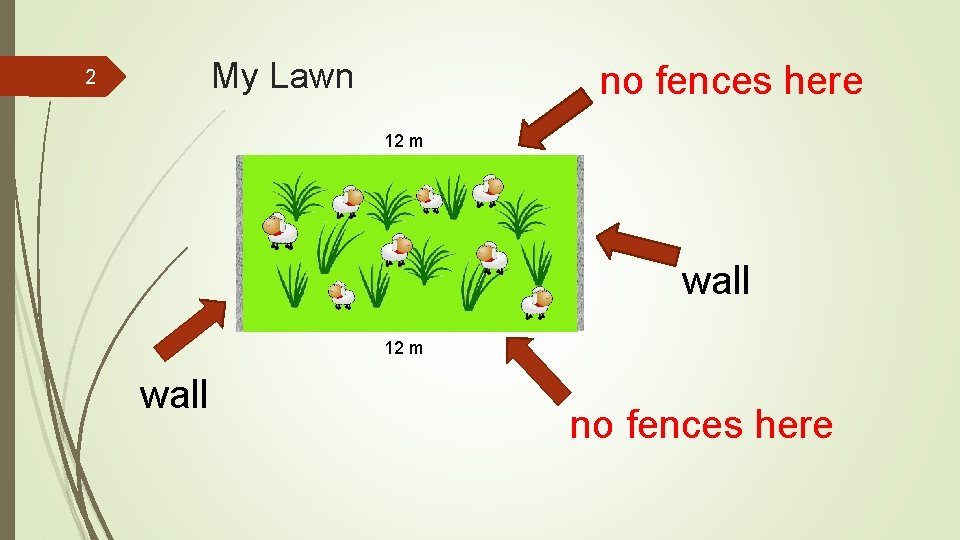My Lawn 2 no fences here 12 m wall no fences here3 Fence？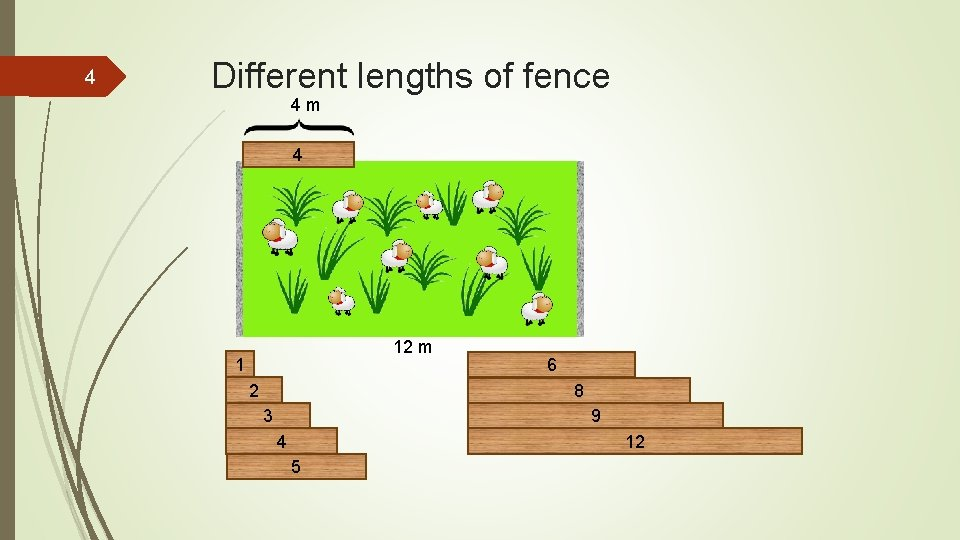4 Different lengths of fence 4 m 4 12 m 1 2 6 8 9 3 12 4 55 What is the length of fence to choose？ 4 4 4 12 m 4 m fence can fit the side, why? 12 ÷ 4 = 3 No remainder We can say: 4 is a factor of 12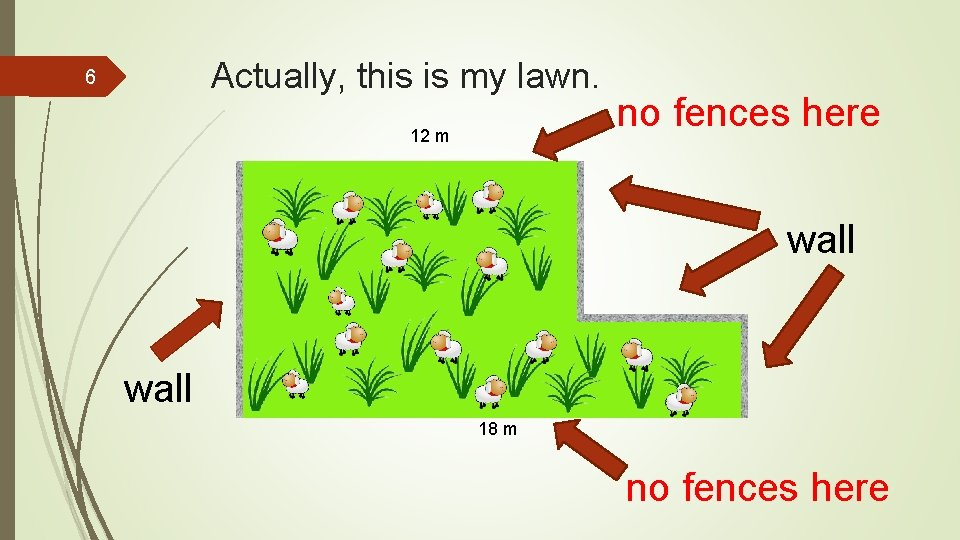Actually, this is my lawn. 6 12 m no fences here wall 18 m no fences here7 What is the length of fence to choose？ 123456 8 9 1213 Someone suggests fences of 13 m long. Is it OK？8 Someone suggests fences of 1 m long. 1 1 1 This is too tiring! Use a longer fence! Fred 1 1 1 1 1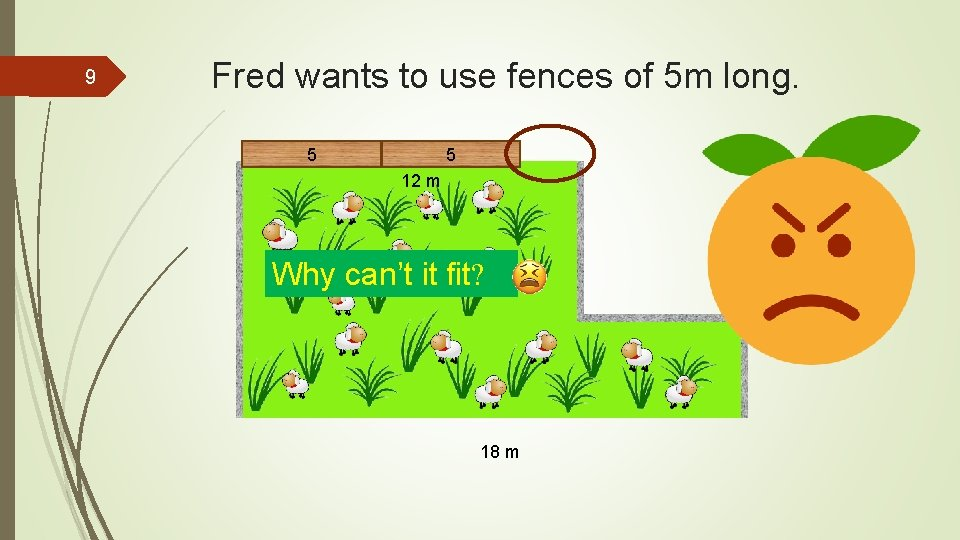9 Fred wants to use fences of 5 m long. 5 5 12 m Why can’t it fit? 18 m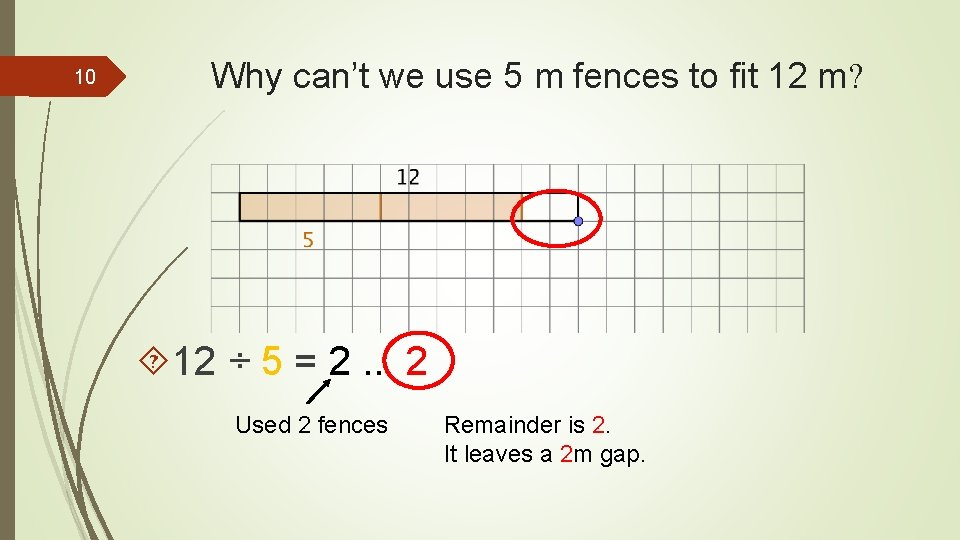10 Why can’t we use 5 m fences to fit 12 m? 12 ÷ 5 = 2. . 2 Used 2 fences Remainder is 2. It leaves a 2 m gap.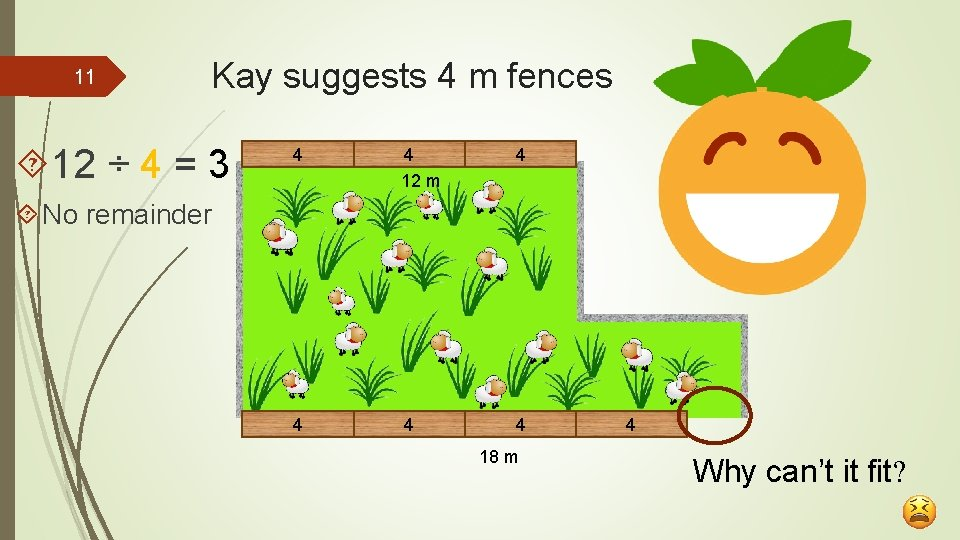11 Kay suggests 4 m fences 12 ÷ 4 = 3 4 4 12 m 4 4 No remainder 18 m 4 Why can’t it fit?12 Why？ Use 4 m fences. Can fit the 12 m side. But not the 18 m side.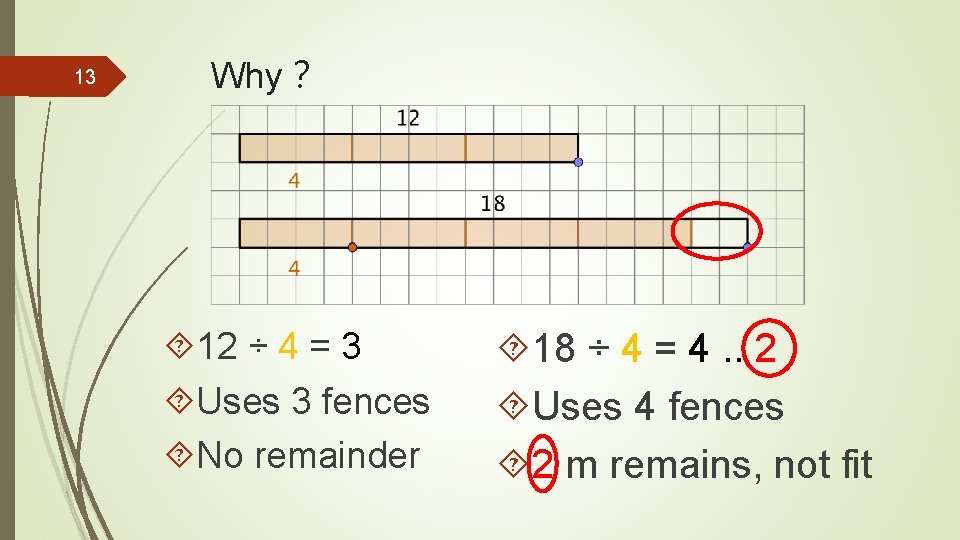13 Why？ 12 ÷ 4 = 3 Uses 3 fences No remainder 18 ÷ 4 = 4. . 2 Uses 4 fences 2 m remains, not fit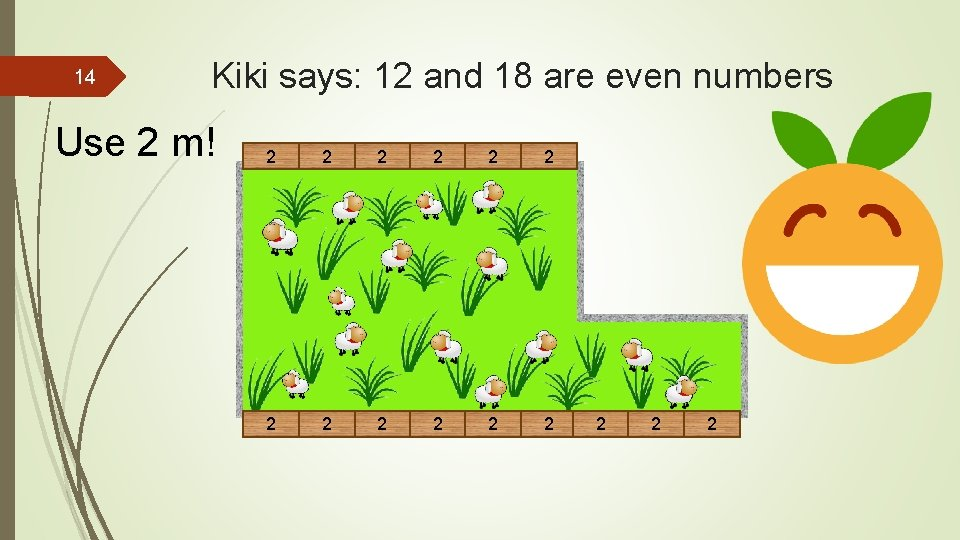14 Kiki says: 12 and 18 are even numbers Use 2 m! 2 2 2 2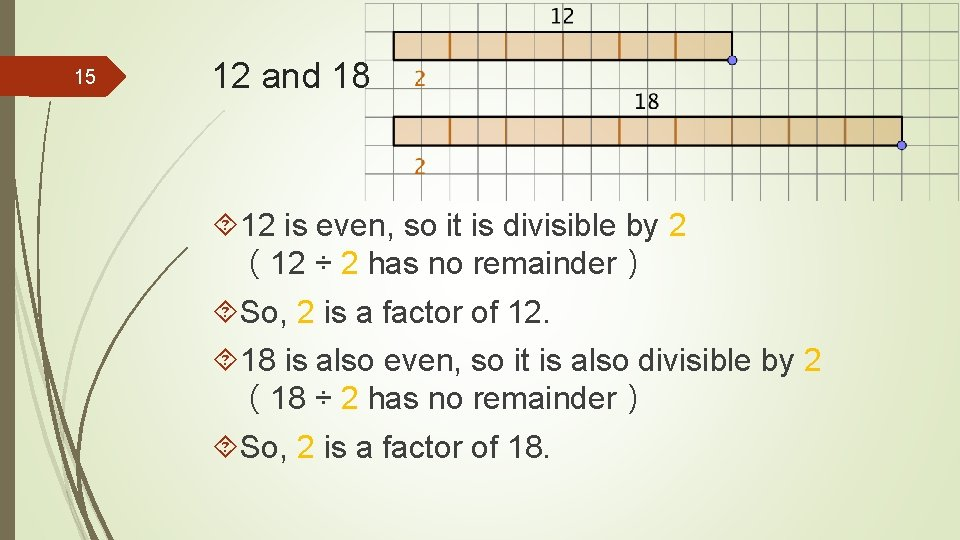15 12 and 18 12 is even, so it is divisible by 2 （12 ÷ 2 has no remainder） So, 2 is a factor of 12. 18 is also even, so it is also divisible by 2 （18 ÷ 2 has no remainder） So, 2 is a factor of 18.12 and 18… 16 2 2 12 and 18 are both divisible by 2 2 2 So, 2 is a factor of 12. and also a factor of 18. 2 is a common factor of 12 and 18. Both have the same factor. 2 2 2 2 2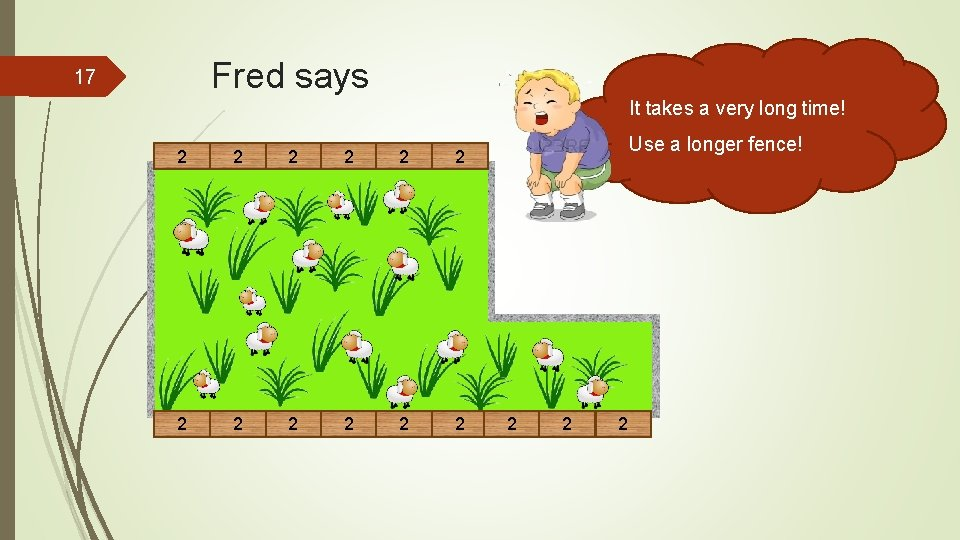Fred says 17 It takes a very long time! 2 2 2 Use a longer fence! 2 2 218 Let’s use the 3 m fences Guess if it fits both sides? Why?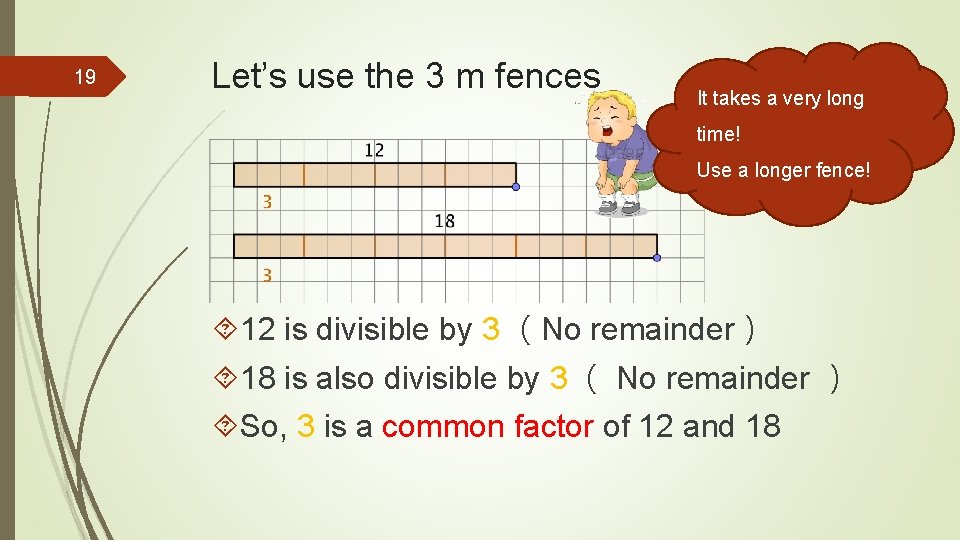19 Let’s use the 3 m fences It takes a very long time! Use a longer fence! 12 is divisible by 3 （No remainder） 18 is also divisible by 3 （ No remainder ） So, 3 is a common factor of 12 and 1820 We have tried 1, 2, 3, 4, 5 m 1 m、2 m、3 m fences can fit Because they are common factors of 12 and 18 4 is a factor of 12, but not a factor of 18 5 is not a factor of 12 and is not a factor of 1821 Let’s use the 6 m fences! Guess if it fits both sides? Why?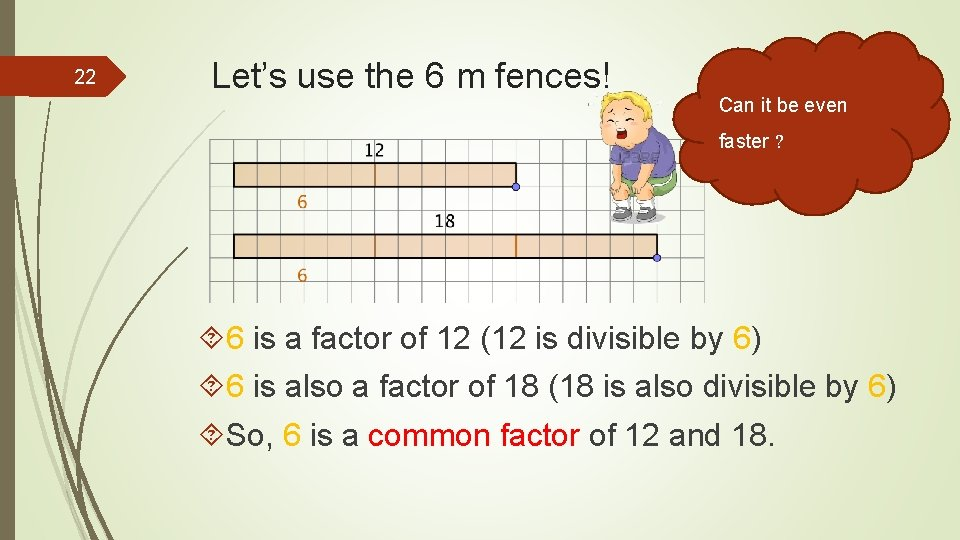22 Let’s use the 6 m fences! Can it be even faster ? 6 is a factor of 12 (12 is divisible by 6) 6 is also a factor of 18 (18 is also divisible by 6) So, 6 is a common factor of 12 and 18.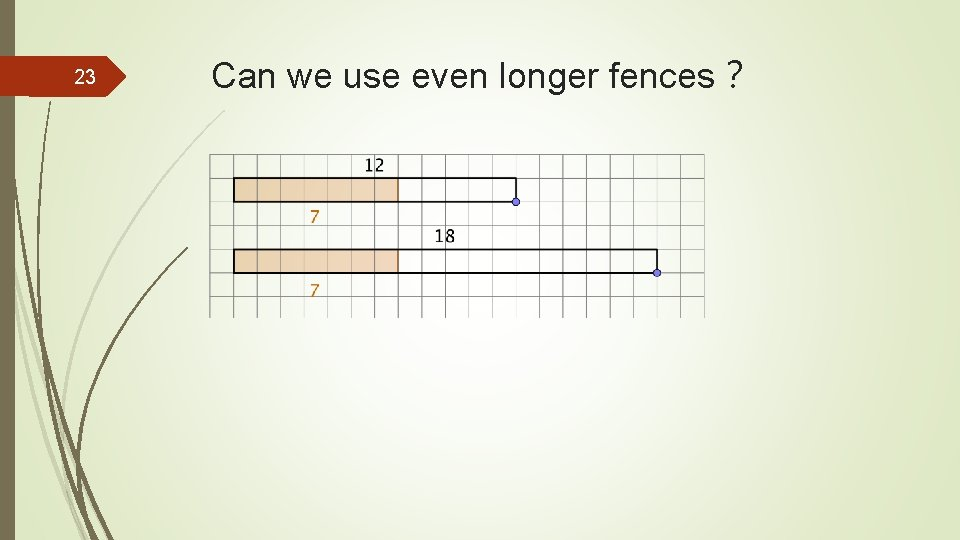23 Can we use even longer fences？24 Can we use even longer fences？ 7 is not a factor of 12, and not a factor of 1825 Can we use even longer fences？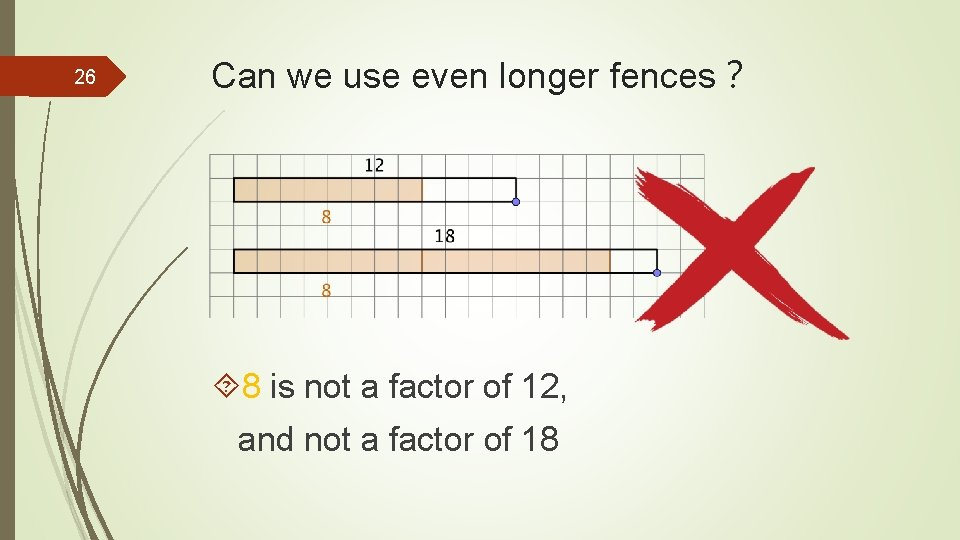26 Can we use even longer fences？ 8 is not a factor of 12, and not a factor of 18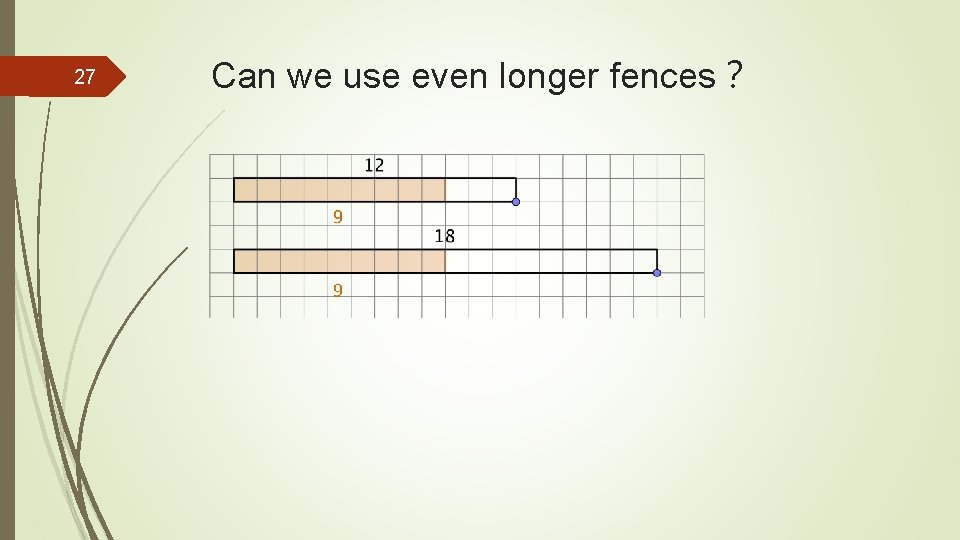27 Can we use even longer fences？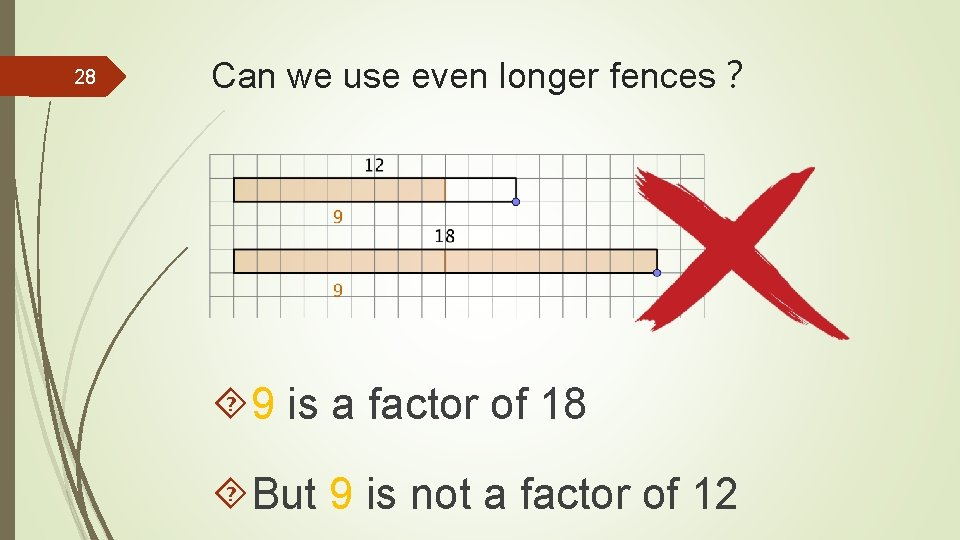28 Can we use even longer fences？ 9 is a factor of 18 But 9 is not a factor of 12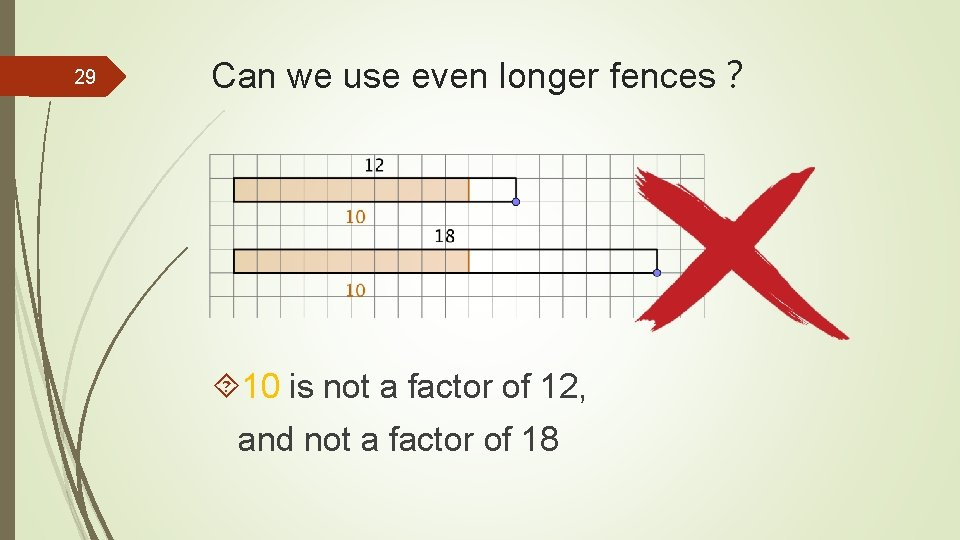29 Can we use even longer fences？ 10 is not a factor of 12, and not a factor of 1830 Can we use even longer fences？ 11 is not a factor of 12, and not a factor of 1831 Can we use even longer fences？ 12 is a factor of 12, But not a factor of 18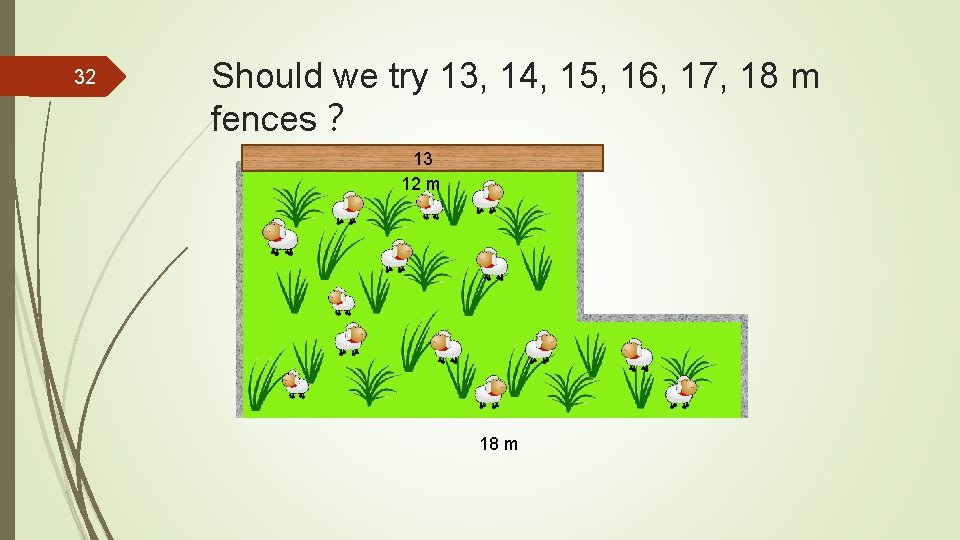32 Should we try 13, 14, 15, 16, 17, 18 m fences？ 13 12 m 18 m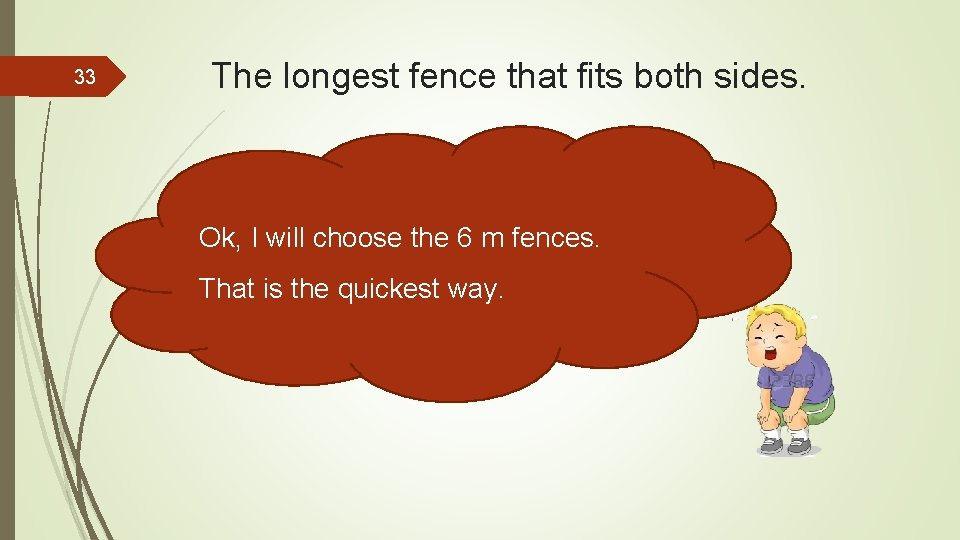33 The longest fence that fits both sides. Ok, I will choose the 6 m fences. That is the quickest way.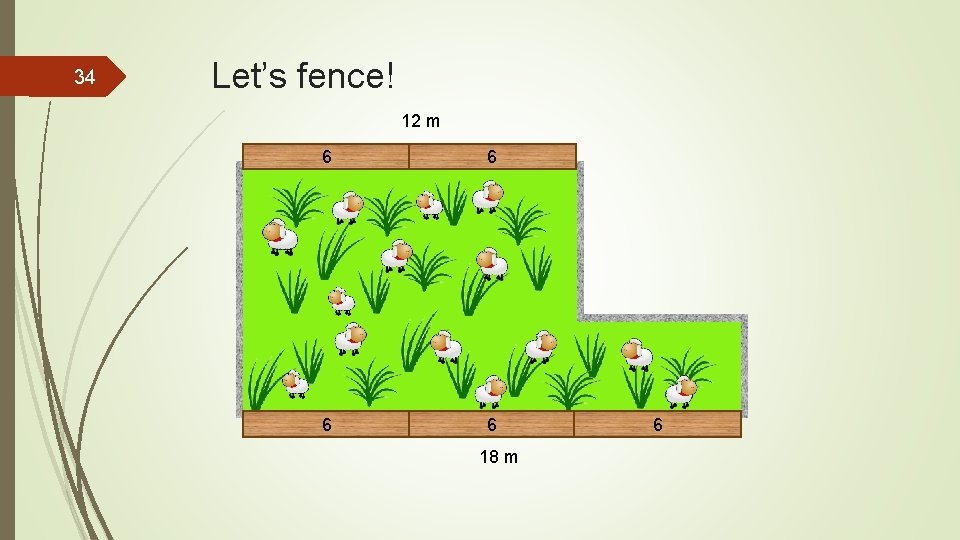34 Let’s fence! 12 m 6 6 18 m 635 Summary Factors of 12 are 1, 2, 3, 4, 6, 12 Factors of 18 are 1, 2, 3, 6, 9, 18 Common factors are 1, 2, 3, 6 Among them, the common factor is 6 Highest 6 is the Highest common factor (H. C. F. ) of 12 and 18